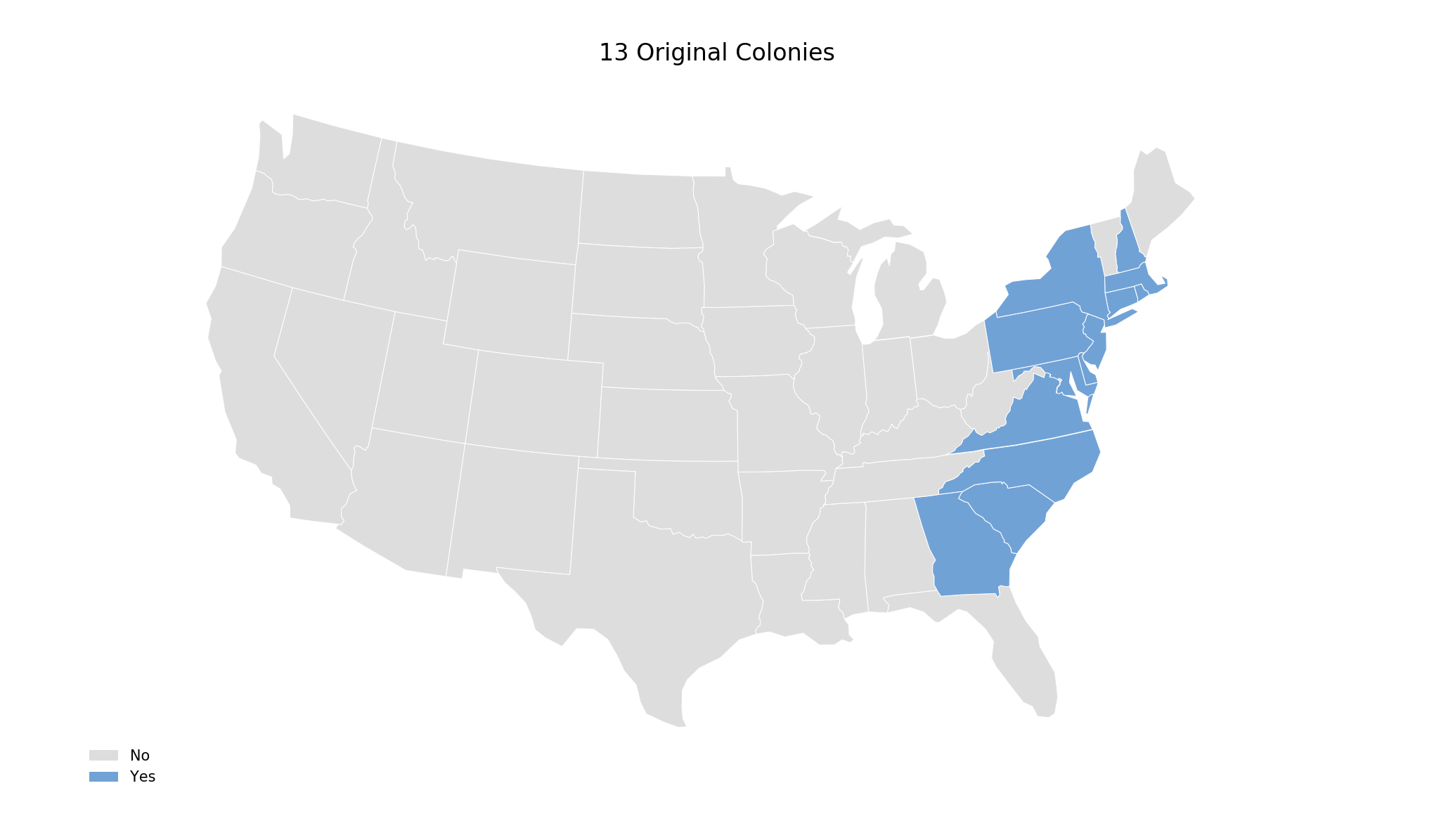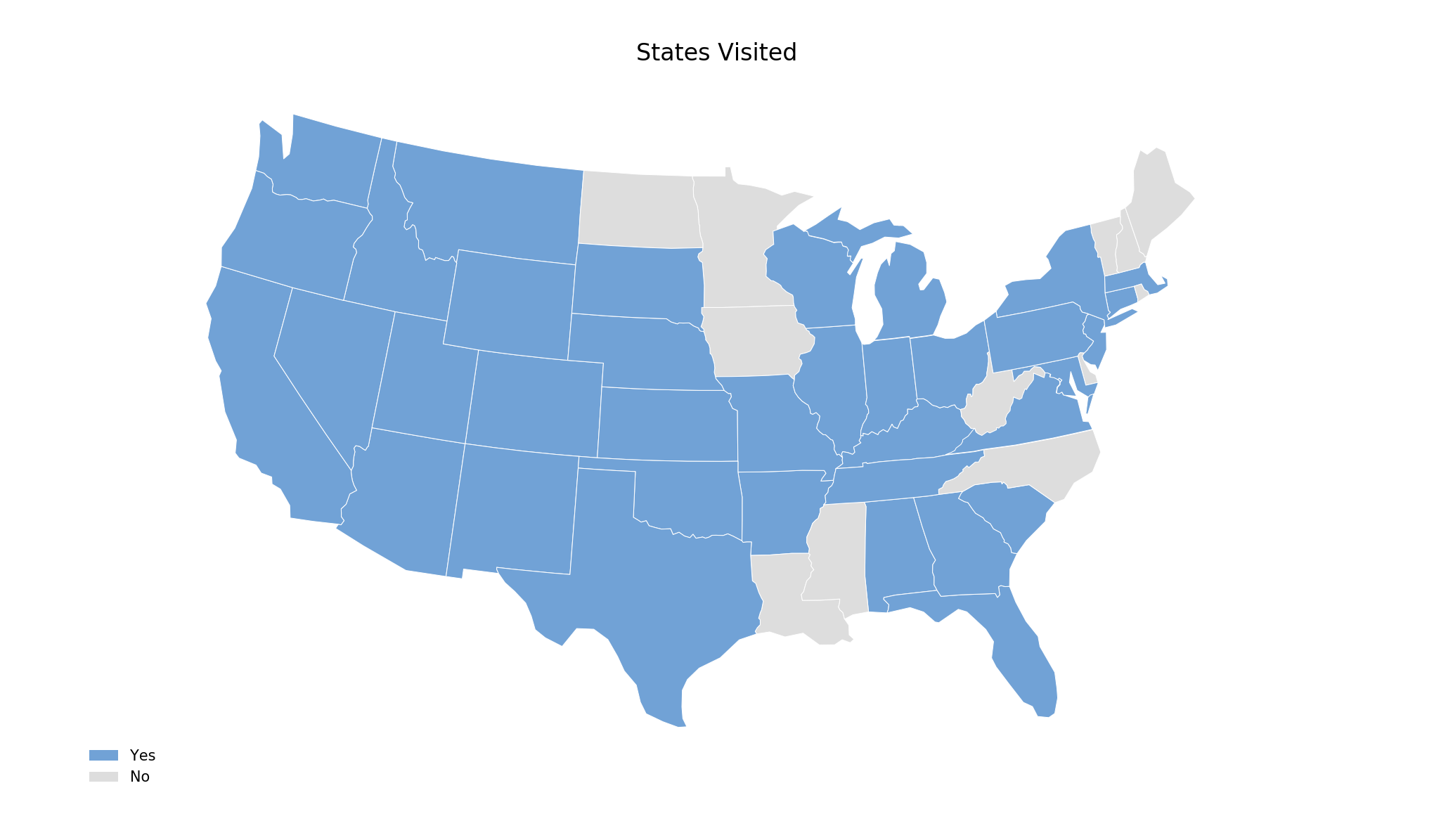# Highlighting States With CartopyHere is how to highlight select US States with Cartopy. (You can also highlight select countries with Cartopy.)

Define which states to highlight in states.csv, and run states.py:

import matplotlib.patches as mpatches
import matplotlib.pyplot as plt
import shapely.geometry as sgeom

import cartopy.crs as ccrs

import pandas as pd

# derived from this example: https://scitools.org.uk/cartopy/docs/v0.15/examples/hurricane_katrina.html

def plot_states(df,projection,colors,annotation,title,edgecolor):

ax = plt.axes([0, 0, 1, 1],
projection=projection)
ax.background_patch.set_visible(False)
ax.outline_patch.set_visible(False)
ax.set_extent([-125, -66.5, 20, 50], ccrs.Geodetic())

category='cultural', name=shapename)

values = list(df[title].unique())

for state in states:
attribute = 'name'
name = state.attributes[attribute]

# get classification
try:
classification = df.loc[state.attributes[attribute]][title]
except:
pass

facecolor=(colors[values.index(classification)]),
label=state.attributes[attribute],
edgecolor='#FFFFFF',
linewidth=.25)

# legend
import matplotlib.patches as mpatches
handles = []
for i in range(len(values)):
handles.append(mpatches.Rectangle((0, 0), 1, 1, facecolor=colors[i]))
plt.legend(handles, values,
loc='lower left', bbox_to_anchor=(0.025, -0.0),
fancybox=True, frameon=False, fontsize=5)

# annotate
ax.annotate(annotation, xy=(0, 0),  xycoords='figure fraction',
xytext=(0.0275, -0.025), textcoords='axes fraction',
horizontalalignment='left', verticalalignment='center', fontsize=4,
)

plt.title(title, fontsize=8)

title = title+'.png'
print('Saved: {}'.format(title))

def main():

# States Visited
projection = ccrs.LambertConformal()
title = 'States Visited'
colors = ['#71a2d6','#DDDDDD']
annotation = ''
plot_states(df,projection,colors,annotation,title,edgecolor='white')

# 13 Original Colonies
projection = ccrs.LambertConformal()
title = '13 Original Colonies'
colors = ['#DDDDDD','#71a2d6']
annotation = ''
plot_states(df,projection,colors,annotation,title,edgecolor='white')

print('Done.\n')

if __name__ == '__main__':
main()


For example, here are the 13 original colonies:Or you can make a map of all states you have visited: